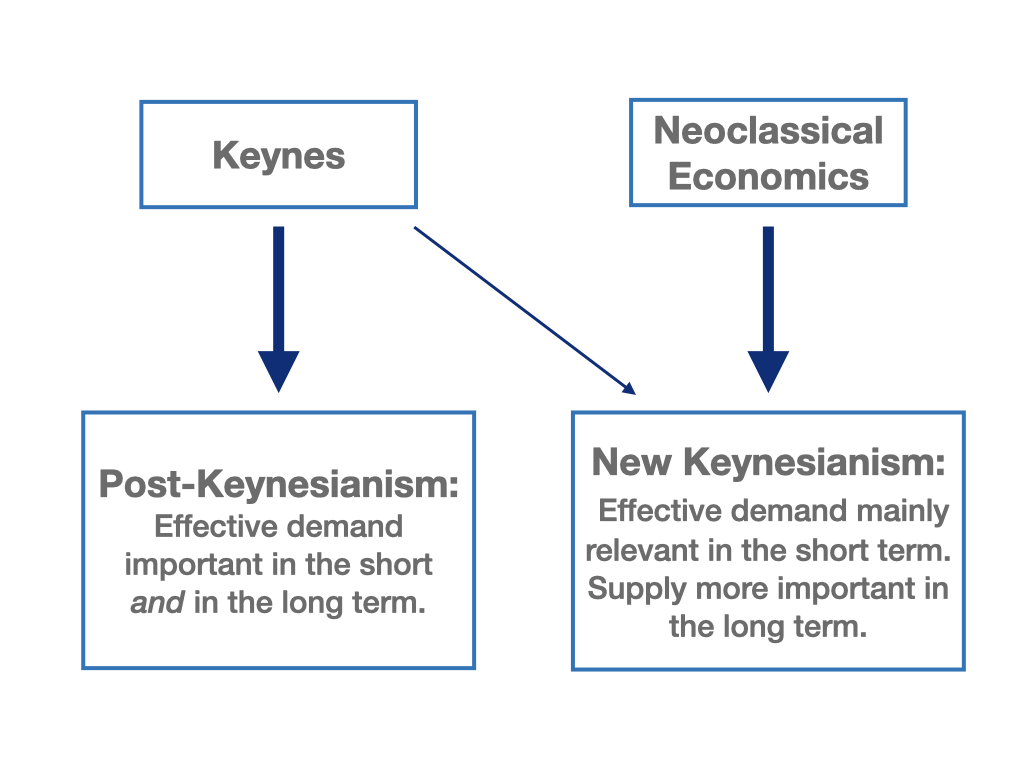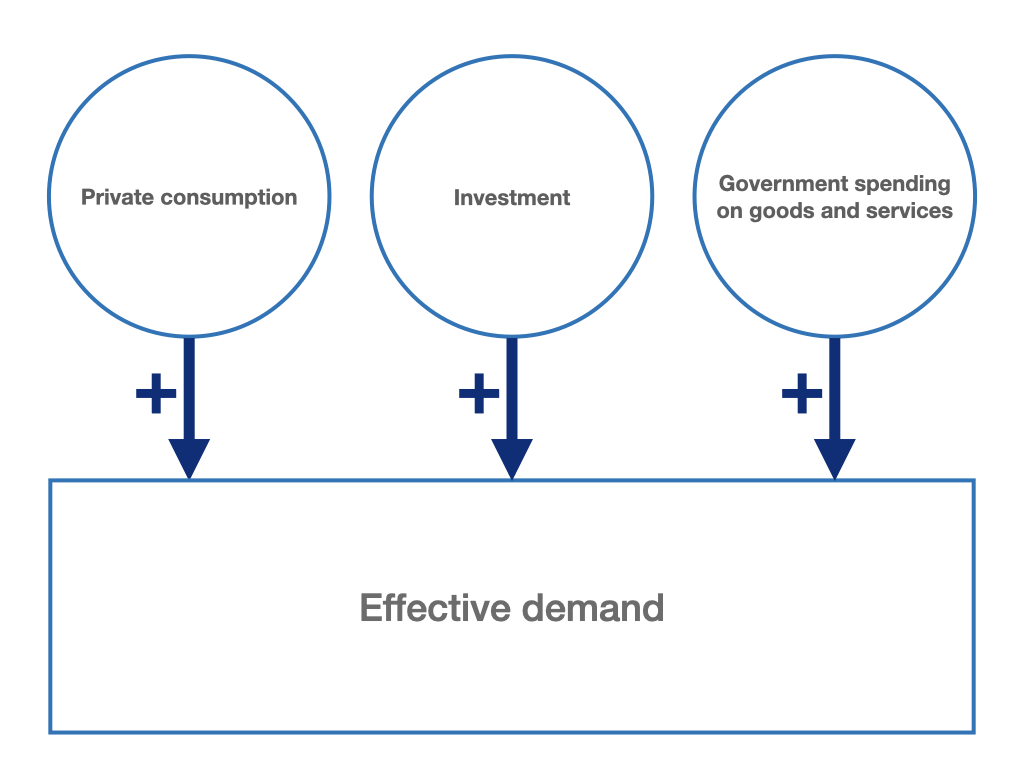# Chapter 2 Aggregate demand: introduction

In this and the following chapters, we will deal with the aggregate demand side of our model economy. Many economists nowadays agree that effective demand is the determining aggregate economic variable, at least in the short run. Fluctuations in aggregate demand lead to adjustment processes on the production, income and expenditure side of the economy and thus trigger business cycle movements. However, demand itself depends on a large number of factors, as it is the sum of the spending decisions of all actors in an economy. Aggregate effective demand is thus the sum of spending by households, firms and the government. In open economies with trade relations with foreign countries, foreign demand for domestic goods, i.e. exports, is added, while domestic demand for foreign goods, i.e. imports, is subtracted.

Why “effective” demand?

We speak of “effective” demand when the demand for a product is not only hypothetically present (e.g. the principal desire to demand a product), but also effectively felt in the goods market through spending decisions. John Maynard Keynes (1936, chap. 3) described effective demand as the demand expected by firms for their products in the goods market. It therefore forms a basis for firms’ production planning.

The demand side of a macroeconomic model for a closed economy thus revolves around the aggregate spending decisions of households, firms and the government and how these decisions affect macroeconomic activity. In the first step of our analysis, we focus in particular on how effective demand can be determined and how it affects aggregate output and aggregate income. A change in the demand for goods and thus in the volume of output then in turn affects the demand for labour in an economy. Thus, effective demand is also of immense importance for employment. Whether an economy is close to full employment or is instead plagued by unemployment depends heavily, at least in the short run, on effective demand in the goods market.

The role of demand: disagreement in macroeconomics

As we will see later, the medium- to long-term role of demand in the state of an economy is highly contested in macroeconomics. A rough division of the different views can be made along two basic macroeconomic paradigms, Keynesian and neoclassical.

The delineation of these basic approaches in macroeconomics can sometimes cause confusion, as hybrids of the Keynesian and of the neoclassical paradigm also exist. In this book, we distinguish between traditional Keynesianism, or post-Keynesianism, and new Keynesianism, which is heavily influenced by the neoclassical theory. While traditional Keynesian and post-Keynesian economists attribute a decisive role to effective demand in the short and long run, new Keynesian and especially neoclassical economists see long-term economic development as mainly determined by supply-side factors. Nevertheless, both demand and supply play an important role in most macroeconomic theories.

The aim of this book is, among other things, to show how exactly the post-Keynesian and the new Keynesian views of the determinants and macroeconomic importance of effective demand differ. However, this will only become clear in its entirety in later chapters, when we compare a new Keynesian and a post-Keynesian model of supply and demand. Before that, we want to shed light on the short-run determinants of demand, output, employment and the price level, i.e. inflation.

Further literature comparing different macroeconomic paradigms can be found, for example, in Heine and Herr (2013), Snowdon and Vane (2005) and Froyen (2002).Figure 2.1: Keynes vs. the Neoclassicals.

We can set up the definition of effective demand with more precise reference to the various expenditures in the economy. This allows us to capture the demand side of our economy and its influencing variables in a simple model. The total real demand for goods and services, $$Y_N$$, is the sum of the real expenditures made by the individual actors in an economy (households, firms, government) on domestically produced goods and services. By definition, it is identical to gross domestic product (GDP) according to the expenditure account, which in a closed economy can be calculated from the sum of aggregate real consumption, $$C$$, real gross investment expenditures, $$I$$, and real government expenditures, $$G$$:

$\begin{equation} Y_N = C + I + G \tag{2.1} \end{equation}$Figure 2.2: Components of effective demand in a closed economy.

In reality, equation (2.1) and the corresponding representation in the figure above only apply to the world economy as a whole since it is a closed economy. If we do not consider the entire planet Earth but only individual regions or countries, we must always assume an open economy. In this case, exports ($$X$$), are added to a country’s effective domestic demand as foreign demand for domestic goods, and imports, ($$M$$), are subtracted as domestic demand for foreign goods. Exports minus imports are referred to as net exports, $$(X-M)$$, as we have seen in chapter 1. The aggregate effective demand of an open economy is then obtained as:

$\begin{equation} Y_N = C + I + G + (X-M) \tag{2.2} \end{equation}$

However, we will initially limit ourselves here to closed economy modeling. This allows us to focus first on the basic mechanisms and cause-effect relationships of our models.

For the closed economy in equation (2.1), aggregate private consumption, private investment and government spending on goods and services form the individual components of aggregate demand. In the following chapters, we will look at these demand components in detail and discuss some of their key determinants. From the various factors influencing consumption, investment, and government spending, we will then first derive simple behavioral equations for $$C$$ and $$I$$ that do not yet contain macroeconomic interactions. In chapter 7, we will then return to equation (2.1) to develop an initial macroeconomic model of the entire demand side of a closed economy, which will then include the interactions between the various demand components (e.g., via the change in income). Here, we will consider government spending also as a policy variable.

## Further reading on chapter 2

Textbooks:

### Literature

Froyen, R. T. 2002. Macroeconomics: Theories and Policies. 7th (or Later) Edition. Upper Saddle River, NJ: Prentice Hall.
Hein, E. 2023. Macroeconomics After Kalecki and Keynes: Post-Keynesian Foundations. Cheltenham: Edward Elgar Publishing, forthcoming.
Heine, M., and H. Herr. 2013. Volkswirtschaftslehre: Paradigmenorientierte Einführung in Die Mikro- Und Makroökonomie. 4. Aufl. München: Oldenbourg.
Keynes, J. M. 1936. The General Theory of Employment, Interest, and Money. London: Palgrave Macmillan.
Snowdon, B., and H. R. Vane. 2005. Modern Macroeconomics: Its Origins, Development and Current State. Cheltenham, UK: Edward Elgar Publishing.